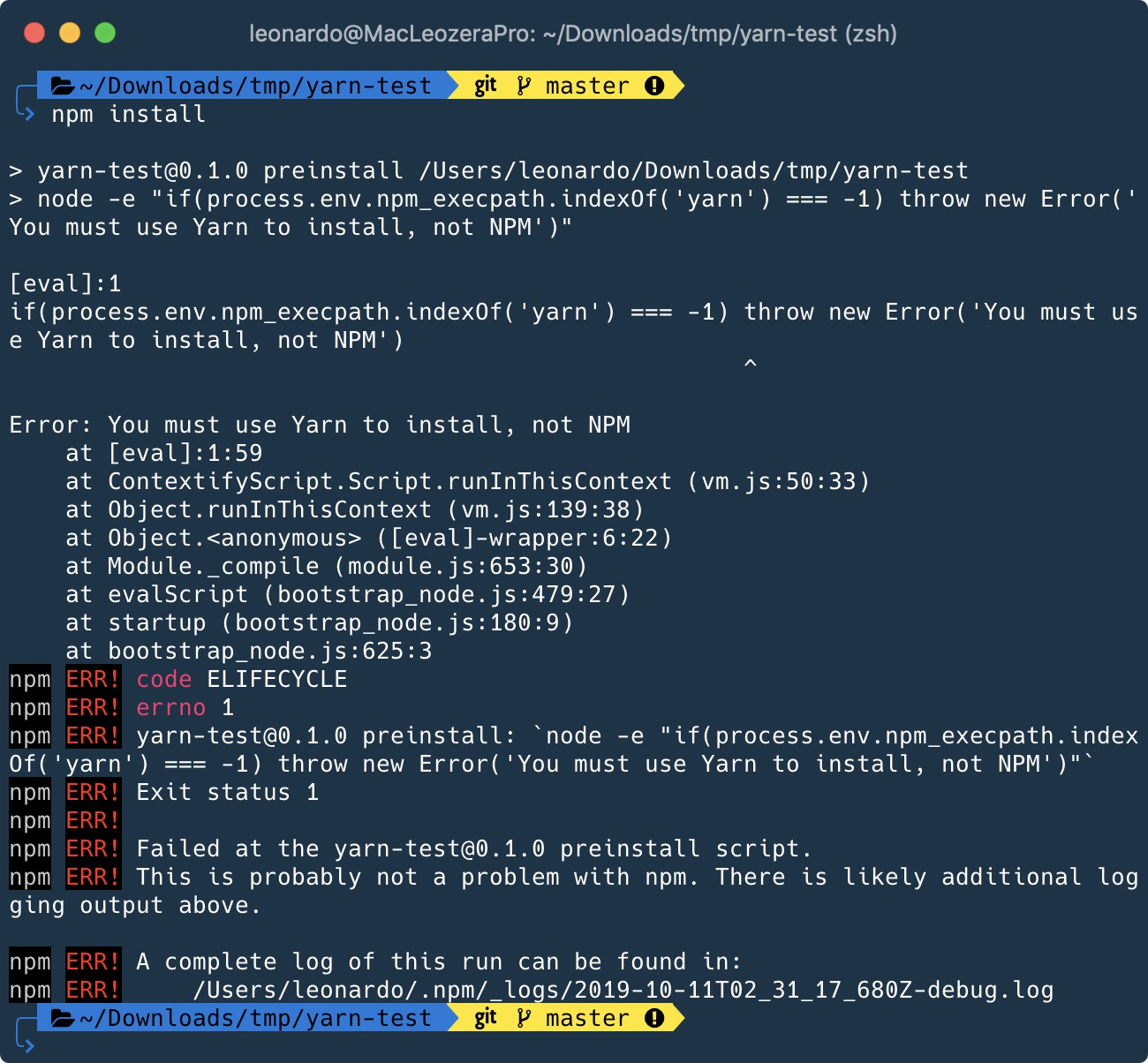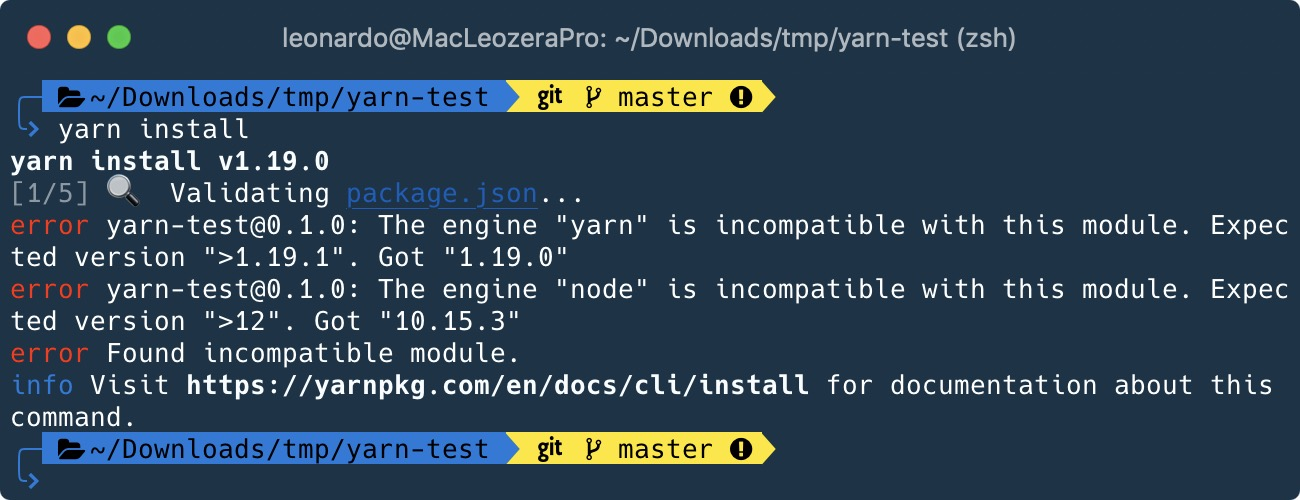javascript

# Forcing the usage of yarn (and at a specific version)self.__wrap_b=(e,n,t)=>{t=t||document.querySelector(`[data-br="\${e}"]`);let c=t.parentElement,r=v=>t.style.maxWidth=v+"px";t.style.maxWidth="";let o=c.clientWidth,l=c.clientHeight,a=o/2,i=o,d;if(o){for(;a+1<i;)d=~~((a+i)/2),r(d),c.clientHeight===l?i=d:a=d;r(i*n+o*(1-n))}t.__wrap_o||(t.__wrap_o=new ResizeObserver(()=>{self.__wrap_b(0,+t.dataset.brr,t)})).observe(c)};self.__wrap_b(":Rhd6:",1)

People and organizations often have preferences for a specific package manager. At work, we decided to use Yarn due to emoji support (jk) but how to keep everybody using Yarn?

We can use the preinstall hook to check if the user run `npm install`or `yarn install`. Here is one example:

package.json
``````"scripts": {
"preinstall": "node -e \"if(process.env.npm_execpath.indexOf('yarn') === -1) throw new Error('You must use Yarn to install, not NPM')\"",
}``````

If you run `npm install`:If you want to ignore the checking (CI environment for instance), use the `--ignore-scripts` option:

``````npm install --ignore-scripts
``````

Moreover, you can use the engines option of NPM to force a specific version of Node, and/or Yarn. Here is an example:

package.json
``````"engines": {
"yarn": ">1.19.1",
"node": ">12"
},``````## Interactions

### Webmentions

Like this content? Buy me a coffeeor share around: